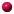#Newton's Laws

## Newton's First Law

• An object at rest will remain at rest
• An object in motion will stay in motion - in a straight line, at the same speed
• As long as no force is applied (more accurately, no unbalanced force).

## Newton's Second Law

• An object's acceleration is proportional to the force applied to it.
• The force to accelerate an object is proportional to the object's mass.
• In equation form, if we call the force "F", the object's mass "m" and the acceleration "a", then Newton's Second Law is simply

F = m * a

which is the most famous form of this fundamental principle of physics.Go To How This Works

## Newton's Third Law

"For every action, there is an equal and opposite reaction."Back to Rocket Simulation Page

## Questions

Your questions and comments regarding this page are welcome. You can e-mail Randy Culp (Tripoli #6926) for inquiries, suggestions, new ideas or just to chat.
Updated 24 August 2008